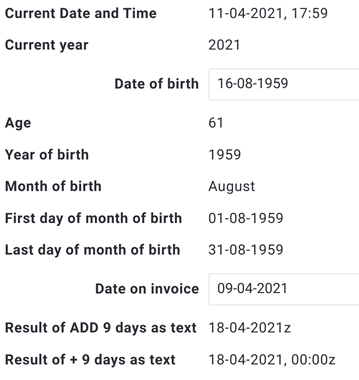# Conversions between numbers, texts and dates

Good to know before you start: different calculations with dates result in different formats. The format of the result of a calculation with days depends on the type of calculation.

1. When you add or subtract days with the + or , you get a date in the format: dd/mm/yyyy, hh:mm.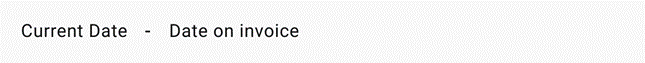2. When you divide the result by one day, it will become a number: n.000000, where n is the number of days.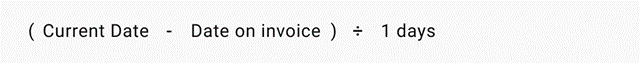3. When you use the Triggre functions to add, you get a date without the time: dd/mm/yyyy.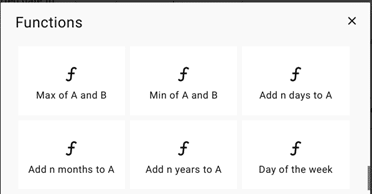## Strip the time from a date

In a flow part, a calculation with dates might result in a date-time value. If you include this in an email, it will look like this: pay before 09/02/2022 03:22. This doesn’t look great, so how to fix this? Convert the result to a text. From there on, you can isolate the date and use that in the data flow.

1. Combine the result with nothing (in With, select Specific value, enter nothing and press Next).The result is a text in the format dd/mm/yyyy hh:mm. For a date, you only need the first ten characters.
2. Isolate the date from the text: cut the first 10 characters.3. Be aware: the result is what you cut (the date), not what you didn’t cut (the time).

## Calculations with dates

For some calculations you need an assisting data item. The steps below explain how to create an assisting data item.
1. Create the data item Year.
2. Allow import.
3. Add four properties: year (number, no decimals), first day (date), last day (date) and year as text.
4. Create the data item Month.
5. Allow import.
6. Add three properties: name (text), number (number, no decimals) and number as text.
7. Create the data item Text to number.
8. Allow import.
9. Add two properties: text (text) and number (number, no decimals).
10. For all three data items: create a user flow to manage the data item, add the user flow to Appearance and publish.
11. Create an Excel file with three sheets: year, month and text to number or make three separate Excel files.
12. Fill the sheets.
13. Don’t forget to add [ID] to a unique column name.
14. Import the Excel file(s) using Unique data.

## Put all date calculations in one flow part

It can be smart to use one flow part for all date conversions: you have all calculations at hand and a user flow needs to call only one flow part.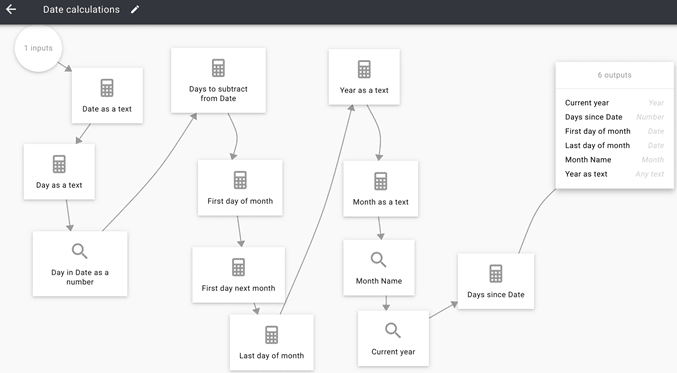Input
The input is a Date.

Convert the date to a text
The first calculation in the Flow part converts this date to a text.
This is the source of most of the calculations “downstream”.
The result will be dd/mm/yyyy, name it Date as a text.## First day of the month

When you isolate the day, the result will be a text. Using data item Month, find the matching number of the day. Subtract (this number – 1) from the input. This will give you the first day of the month. Add one month, subtract one day and you get the last day of the month.

1. Day as a text: cut the first 2 characters of Date as a text to isolate the day.2. Day in Date as a number: look up the matching number in data item Text to number.3. Days to subtract from Date: define the number of days that will be added to the date: the date minus one, and that as a negative number.An example: if the day of Date = 12, you must subtract 11 in order to arrive at day 1, the first day of the month. This is the same as adding -11. The function below can only add, that’s why you have to make it a negative number.
4. First day of month: addthis value to date. This will result in the first day of the month as a date.## The last day of a month

Add a month to the first day of the month and then add -1 day to it. That will give you the last day of the month as a date.
1. First day next month:2. Last day next month:## Year as a text

When you want to display or email a calculated year, Triggre will show it as a number (y,yyy). This doesn’t look nice. Just convert the number to a text and use the result.## The name of the month

First isolate the month number as a text. Then look up the month in data item Month. This will provide you with the name of the month.
1. Isolate the month: position 4 and 5. The result is the number of the month as a text.2. Look up the month: look up the month matching the number of the month.## The current year

You need the data item Year to find the year.
1. Look up the Year in which the Current date fits:## Number of days since a specific date

If you need to know how many days have passed since something happened, you can use this calculation. The result is a number with 6 decimals.## Remove the decimals from a number

1. To strip the decimals from a number, convert the number to a text.
2. Remove the decimal point and everything to the right of it.
Number as a text:Make sure that you enter nothing in the specific value behind WITH.

Determine the amount of digits to the left of the decimal point (n).

There are six decimals behind the decimal point.

This means that if you delete the (1 decimal point + 6 decimals) = 7 most right characters from the length of the text, you get the number as an integer.

An example:
• The number is 123.123456
• The number as a text becomes: 123.123456
• The length is 10 characters
• n = 10 – 7 = 3
• CUT 123.123456 FROM 1 TO 3 results in 123
The calculation of n:Isolate the number (the integer):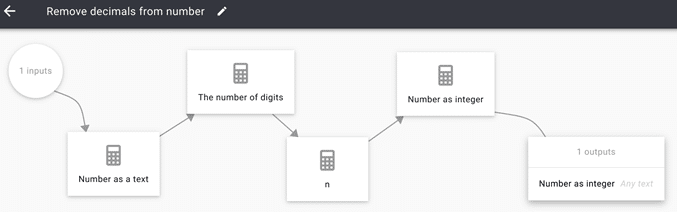## Examples